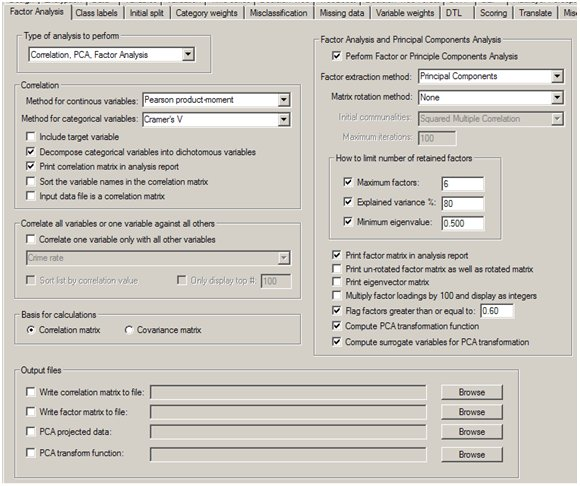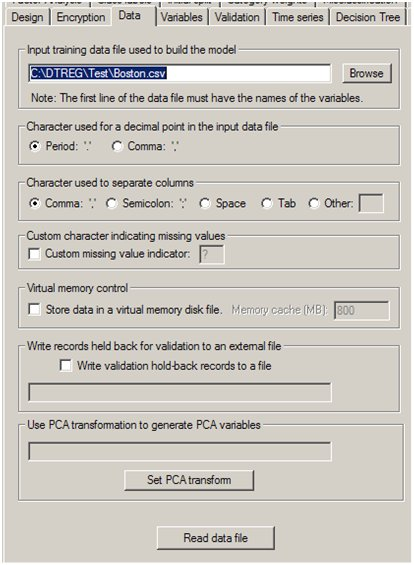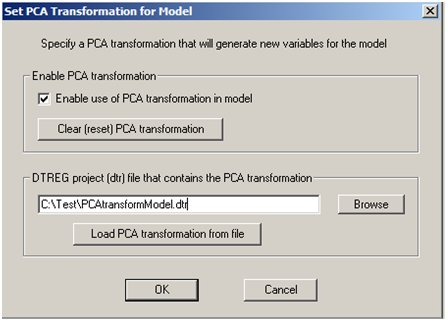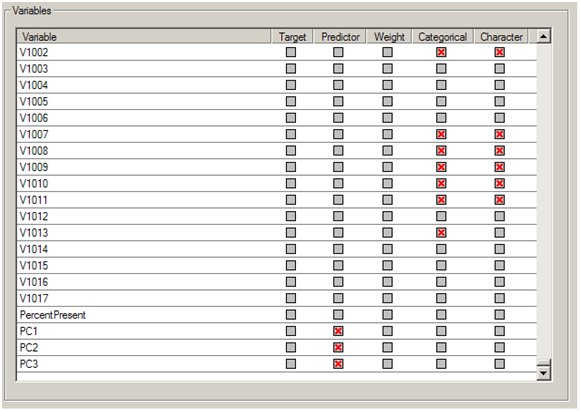# PCA Transformations

## Principal Component Transformations

Principal components are weighted, linear combinations of the variables, and the principal components are ordered in decreasing order of explained variance. It is possible to generate new variables whose values are computed using the eigenvectors. For example, a new variable, PC1, could be computed for each set of variable values using the formula:

PC1 = a11X1 + a12X2 + … + a1nXn

Then this computed variable (PC1) can be used in a predictive model instead of the original variables. Since the principal components (and eigenvectors) are ordered in decreasing order of explained variance, it is often possible to use fewer principal component variables than original variables. For example, the following table taken from a DTREG report shows the percent of total variance explained by each principal component and the cumulative amount explained:

```Factor  Eigenvalue  Variance %  Cumulative %       Scree Plot
------  ----------  ----------  ------------  --------------------
1      6.12685    47.130       47.130     ********************
2      1.43328    11.025       58.155     ****
3      1.24262     9.559       67.713     ****
4      0.85758     6.597       74.310     **
5      0.83482     6.422       80.732     **
6      0.65741     5.057       85.789     **
7      0.53536     4.118       89.907     *
8      0.39610     3.047       92.954     *
9      0.27694     2.130       95.084     *
10      0.22024     1.694       96.778
11      0.18601     1.431       98.209
12      0.16930     1.302       99.511
13      0.06351     0.489      100.000
```

There were 13 original variables, but the cumulative effect of using only the first five principal components accounts for 80.732% of the variance.

One word of caution: principal components are formed from a linear combination of the variables. If the variables are related in a nonlinear manner, the principal components will not correctly reflect the relationship.

The Enterprise Version of DTREG contains features to (1) compute principal component transformations, (2) use the PCA transformations to convert the input data to PCA transformed values, and (3) use PCA transformation functions computed in one model to automatically generate new PCA variables in a subsequent model.

Here are the steps in computing PCA transform functions and then using them to generate PCA variables in a subsequent model.

1. Perform a PCA analysis, select the criteria to determine how many principal components will be stored, and check the option “Compute PCA transformation function” on the PCA properties page.2. After the PCA analysis has been performed, save the generated model to a DTREG project file (.dtr file).
3. Open or create a new project in which you want to use the PCA transformation.
4. On the Data property page for the new model, click the button “Set PCA transform”.5. A popup screen will appear looking like this:6. Check the box “Enable use of PCA transformation in model”, specify the name of the DTREG project file contain the previously-computed PCA transformation, then click the “Load PCA transformation from file” button. DTREG will read the project file containing the PCA transformation function and attach the PCA transformation function to this project. DTREG will report if the PCA transformation was found in the auxiliary project and successfully attached to this project:7. Once the transformation has been read from the auxiliary project file and bound to this model, the auxiliary project file is no longer needed. The PCA transformation function becomes part of the new project, and it will be stored with the new project file. If surrogate variables were computed with the PCA transformation, they also will become part of the new model, and they will be used to handle missing values going into the PCA transformation.
8. After binding a PCA transformation function to the model, new variables will appear in the list of variables on the Variables Property Page with names PCn where nis the principal component number.9. You can then use these variables as predictors in the new model. The PCA variables are also available for predicting values using the Score Function. If you use the DTREG COM DLL component, the PCA transformations will be applied to the input data for computing predictions. If you use DTL with PCA transformations, variables created by DTL may be used as inputs to the PCA transformation function, but the PCA variables created by the transformation are not available to the DTL program.